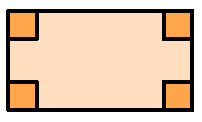### Home > CC2 > Chapter Ch1 > Lesson 1.2.7 > Problem1-128

1-128.

Draw an example of each of the following shapes. If you need help, click each one for the glossary information. Homework Help ✎

 a. rectangleHint (a):What are the characteristics of a rectangle?More Help (a):A rectangle has 4 sides and 4 ninety degree angles.Answer (a):b. squareHint (b):What are the characteristics of a square?More Help (b):A square has 4 sides of equal length and 4 ninety degree angles. c. parallelogramHint (c):What are the characteristics of a parallelogram?More Help (c):A parallelogram has 4 sides with two pairs of parallel sides. d. trapezoidHint (d):What are the characteristics of a trapezoid?More Help (d):A trapezoid is a quadrilateral with at least one pair of parallel sides. e. scalene triangleHint (e):What are the characteristics of a scalene triangle?More Help (e):A scalene triangle has no congruent sides. f. right triangleHint (f):What are the characteristics of a right triangle?More Help (f):A right triangle has one right angle.